# 介绍

## 更新历史

• 增加了关于字典游标（DictCusor）的知识和使用技巧（21/04/24）

• 增加了关于事务机制和提交方式的知识和使用技巧（21/04/24）

# 安装

`pip install pymysql`

# 导入

```import time
import pymysql
from hashlib import md5```

# 连接

```try:
cursor = cn.cursor()
except Exception as e:
print(e)
exit()```

# 数据库列表

pymysql没有单独的方法用来获取数据库列表，福哥是通过执行“show databases”语句获取数据库列表信息的。这是一个最简单的查询语句的示例，大家看好了。

```try:
cursor.execute("show databases")
databases = cursor.fetchall()
print("数据库列表")
print("")
for database in databases:
print(database)
print("")
except Exception as e:
print(e)```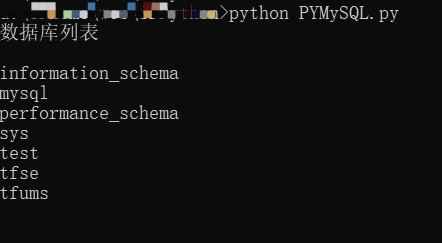# 数据表列表

## 连接

```try:
cn = pymysql.connect(host="192.168.2.168", user="root", password="abcdef", db="tfums", charset="utf8")
cursor = cn.cursor()
except Exception as e:
print(e)
exit()```

## 查询

```try:
cursor.execute("show tables")
tables = cursor.fetchall()
print("数据表列表")
print("")
for table in tables:
print(table)
print("")
except Exception as e:
print(e)```# 插入数据

## 插入数据

```try:
sql = "insert into user (passwd, createDT, userFlag, userState, userName)" \
" values ('%s', now(), %d, %d, '%s')"
params = (md5("123456".encode("utf-8")).hexdigest(), 7, 1, "福哥教pymysql")
print("插入数据")
print("")
cursor.execute(sql % params)
cn.commit()
except Exception as e:
print(e)
cn.rollback()```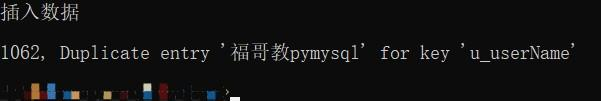## 获取自增列数值

```try:
sql = "insert into user (passwd, createDT, userFlag, userState, userName)" \
" values ('%s', now(), %d, %d, '%s')"
params = (md5("123456".encode("utf-8")).hexdigest(), 7, 1, "福哥教pymysql")
print("插入数据")
print("")
cursor.execute(sql % params)
cn.commit()

print("获取自增列数值")
print("")
print(cursor.lastrowid)
except Exception as e:
print(e)
cn.rollback()```# 更新数据

```try:
sql = "update user set lastLoginDT = now() where userID = %d"
params = (32)
print("更新数据")
print("")
cursor.execute(sql % params)
cn.commit()
effectedRows = cursor.rowcount
print(effectedRows)
except Exception as e:
print(e)
cn.rollback()```# 删除数据

```try:
sql = "delete from user where userID = %d"
params = (32)
print("删除数据")
print("")
cursor.execute(sql % params)
cn.commit()
effectedRows = cursor.rowcount
print(effectedRows)
except Exception as e:
print(e)
cn.rollback()```# 查询数据

```try:
cursor.execute("select * from user order by userID desc")
users = cursor.fetchall()
print("数据表user的数据列表")
print("")
for user in users:
print(str(user) + "@" + user)
print("")
except Exception as e:
print(e)```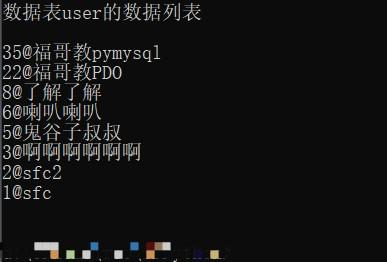# 注意事项

• 连接MySQL和选择数据库可以放到包含文件里面，这样可以实现代码的复用。

• 无论自己的数据库是不是utf-8编码的都设置一下连接编码，毕竟你不能保证运行你的程序的环境一定是utf-8的。

• 尽量不要阻止pymysql抛出异常，有bug我们就解决bug，这样系统才健康！

# 字典游标

## DictCursor

### 示例

```try:
cn = pymysql.connect(host="192.168.2.168", user="root", password="abcdef", db="tfums", charset="utf8")
cursorDict = cn.cursor(pymysql.cursors.DictCursor)
except Exception as e:
print(e)
exit()

try:
cursorDict.execute("select * from user order by userID desc")
users = cursorDict.fetchall()
print("数据表user的数据列表")
print("")
for user in users:
print("")
except Exception as e:
print(e)```# 事务机制

## InnoDB

pymysql相对于MySQL来说也是一个终端，在提交之前也不会受其他终端的更改的影响，所以在MySQL的控制台终端的更改在pymysql里面是查询不到的。

## 事务提交

### 手动提交

```try:
while True:
cursorDict.execute("select * from user order by userID desc")
cn.commit()
users = cursorDict.fetchall()
print("数据表user的数据列表")
print("")
for user in users:
print("")
# wait
time.sleep(10)
except Exception as e:
print(e)```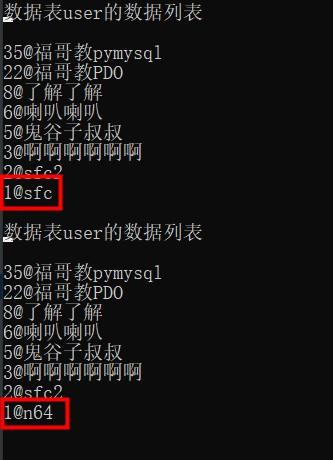### 自动提交

```try:
cn = pymysql.connect(host="192.168.2.168", user="root", password="abcdef", db="tfums", charset="utf8", autocommit=True)
cursor = cn.cursor()
cursorDict = cn.cursor(pymysql.cursors.DictCursor)
except Exception as e:
print(e)
exit()

try:
while True:
cursorDict.execute("select * from user order by userID desc")
users = cursorDict.fetchall()
print("数据表user的数据列表")
print("")
for user in users:
print("")
# wait
time.sleep(10)
except Exception as e:
print(e)```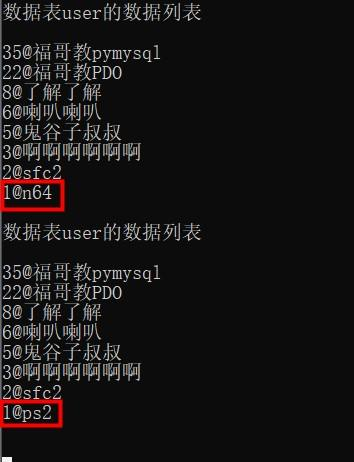212

1626